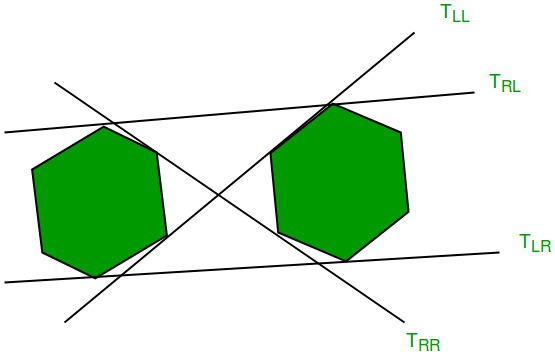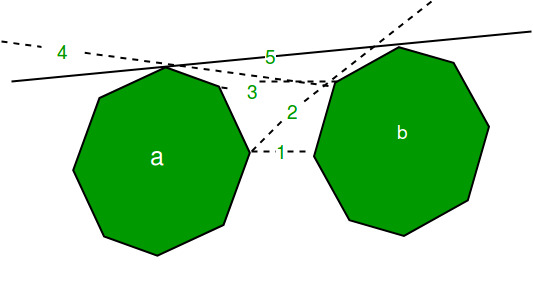# Tangents between two Convex Polygons

• Difficulty Level : Hard
• Last Updated : 22 Jun, 2022

Given two convex polygons, we need to find the lower and upper tangents to these polygons.
As shown in the figure below,andshow upper and lower tangent respectively.Examples:

```Input : First Polygon  : {(2, 2), (3, 3), (5, 2), (4, 0), (3, 1)}
Second Polygon : {(-1, 0), (0, 1), (1, 0), (0, -2)}.
Output : Upper Tangent - line joining (0,1) and (3,3)
Lower Tangent - line joining (0,-2) and (4,0)```

Overview:
Let’s have two convex polygons as shown,For finding the upper tangent, we start by taking two points. The rightmost point of a and leftmost point of b. The line joining them is labelled as 1. As this line passes through the polygon b (is not above polygon b) so we take the anti-clockwise next point on b, the line is labelled 2. Now the line is above the polygon b, fine! But the line is crossing the polygon a, so we move to the clockwise next point, labelled as 3 in the picture. This again crossing the polygon a so we move to line 4. This line is crossing b so we move to line 5. Now this line is crossing neither of the points. So this is the upper tangent for the given polygons.
For finding the lower tangent we need to move inversely through the polygons i.e. if the line is crossing the polygon b we move to clockwise next and to anti-clockwise next if the line is crossing the polygon a.
Algorithm for upper tangent:

```L <- line joining the rightmost point of a
and leftmost point of b.
while (L crosses any of the polygons)
{
while(L crosses b)
L <- L' : the point on b moves up.
while(L crosses a)
L <- L' : the point on a moves up.
}```

Algorithm for lower tangent:

```L <- line joining the rightmost point of a
and leftmost point of b.
while (L crosses any of the polygons)
{
while (L crosses b)
L <- L' : the point on b moves down.
while (L crosses a)
L <- L' : the point on a moves down.
}```

Example :

## CPP

 `// C++ program to find upper tangent of two polygons.``#include``using` `namespace` `std;` `// stores the center of polygon (It is made``// global because it is used in compare function)``pair<``int``,``int``> mid;` `// determines the quadrant of a point``// (used in compare())``int` `quad(pair<``int``,``int``> p)``{``    ``if` `(p.first >= 0 && p.second >= 0)``        ``return` `1;``    ``if` `(p.first <= 0 && p.second >= 0)``        ``return` `2;``    ``if` `(p.first <= 0 && p.second <= 0)``        ``return` `3;``    ``return` `4;``}` `// Checks whether the line is crossing the polygon``int` `orientation(pair<``int``,``int``> a, pair<``int``,``int``> b,``                                 ``pair<``int``,``int``> c)``{``    ``int` `res = (b.second-a.second)*(c.first-b.first) -``              ``(c.second-b.second)*(b.first-a.first);` `    ``if` `(res == 0)``        ``return` `0;``    ``if` `(res > 0)``        ``return` `1;``    ``return` `-1;``}` `// compare function for sorting``bool` `compare(pair<``int``,``int``> p1, pair<``int``,``int``> q1)``{``    ``pair<``int``,``int``> p = make_pair(p1.first - mid.first,``                                ``p1.second - mid.second);``    ``pair<``int``,``int``> q = make_pair(q1.first - mid.first,``                                ``q1.second - mid.second);` `    ``int` `one = quad(p);``    ``int` `two = quad(q);` `    ``if` `(one != two)``        ``return` `(one < two);``    ``return` `(p.second*q.first < q.second*p.first);``}` `// Finds upper tangent of two polygons 'a' and 'b'``// represented as two vectors.``void` `findUpperTangent(vector > a,``                      ``vector > b)``{``    ``// n1 -> number of points in polygon a``    ``// n2 -> number of points in polygon b``    ``int` `n1 = a.size(), n2 = b.size();` `    ``// To find a point inside the convex polygon(centroid),``    ``// we sum up all the coordinates and then divide  by``    ``// n(number of points). But this would be a floating-point``    ``// value. So to get rid of this we multiply points``    ``// initially with n1 and then find the centre and``    ``// then divided it by n1 again.``    ``// Similarly we do divide and multiply for n2 (i.e.,``    ``// elements of b)` `    ``// maxa and minb are used to check if polygon a``    ``// is left of b.``    ``int` `maxa = INT_MIN;``    ``for` `(``int` `i=0; i rightmost point of a``    ``int` `ia = 0, ib = 0;``    ``for` `(``int` `i=1; i a[ia].first)``            ``ia = i;` `    ``// ib -> leftmost point of b``    ``for` `(``int` `i=1; i 0)``            ``inda = (inda + 1) % n1;` `        ``while` `(orientation(a[inda], b[indb], b[(n2+indb-1)%n2]) < 0)``        ``{``            ``indb = (n2+indb-1)%n2;``            ``done = 0;``        ``}``    ``}` `    ``cout << ``"upper tangent ("` `<< a[inda].first << ``","``        ``<< a[inda].second << ``") ("` `<< b[indb].first``        ``<< ``","` `<< b[indb].second << ``")\n"``;``}` `// Driver code``int` `main()``{``    ``vector > a;``    ``a.push_back({2, 2});``    ``a.push_back({3, 1});``    ``a.push_back({3, 3});``    ``a.push_back({5, 2});``    ``a.push_back({4, 0});` `    ``vector > b;``    ``b.push_back({0, 1});``    ``b.push_back({1, 0});``    ``b.push_back({0, -2});``    ``b.push_back({-1, 0});` `    ``findUpperTangent(a, b);` `    ``return` `0;``}`

Output:

`Upper tangent (0,1) (3,3)`

Time Complexity: O(n1 log (n1) + n2 log(n2))
Auxiliary Space: O(1)

Note that the above code only finds upper tangent. We can similarly find lower tangent.
This article is contributed by Aarti_Rathi and Amritya Vagmi and would like to contribute, you can also write an article using write.geeksforgeeks.org or mail your article to review-team@geeksforgeeks.org. See your article appearing on the GeeksforGeeks main page and help other Geeks.